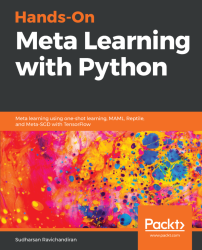•#### Hands-On Meta Learning with Python#### Overview of this book

Meta learning is an exciting research trend in machine learning, which enables a model to understand the learning process. Unlike other ML paradigms, with meta learning you can learn from small datasets faster. Hands-On Meta Learning with Python starts by explaining the fundamentals of meta learning and helps you understand the concept of learning to learn. You will delve into various one-shot learning algorithms, like siamese, prototypical, relation and memory-augmented networks by implementing them in TensorFlow and Keras. As you make your way through the book, you will dive into state-of-the-art meta learning algorithms such as MAML, Reptile, and CAML. You will then explore how to learn quickly with Meta-SGD and discover how you can perform unsupervised learning using meta learning with CACTUs. In the concluding chapters, you will work through recent trends in meta learning such as adversarial meta learning, task agnostic meta learning, and meta imitation learning. By the end of this book, you will be familiar with state-of-the-art meta learning algorithms and able to enable human-like cognition for your machine learning models.
Title PageDedicationContributorsPrefaceFree Chapter
Introduction to Meta LearningFace and Audio Recognition Using Siamese NetworksPrototypical Networks and Their VariantsRelation and Matching Networks Using TensorFlowMemory-Augmented Neural NetworksMAML and Its VariantsMeta-SGD and ReptileGradient Agreement as an Optimization ObjectiveAssessmentsOther Books You May EnjoyIndex## Types of meta learning

Meta learning can be categorized in several ways, right from finding the optimal sets of weights to learning the optimizer. We will categorize meta learning into the following three categories:

• Learning the metric space
• Learning the initializations
• Learning the optimizer

### Learning the metric space

In the metric-based meta learning setting, we will learn the appropriate metric space. Let's say we want to learn the similarity between two images. In the metric-based setting, we use a simple neural network that extracts the features from two images and finds the similarity by computing the distance between features of these two images. This approach is widely used in a few-shot learning setting where we don't have many data points. In the upcoming chapters, we will learn about metric-based learning algorithms such as Siamese networks, prototypical networks, and relation networks.

### Learning the initializations

In this method, we try to learn optimal initial parameter values. What do we mean by that? Let's say we are a building a neural network to classify images. First, we initialize random weights, calculate loss, and minimize the loss through a gradient descent. So, we will find the optimal weights through gradient descent and minimize the loss. Instead of initializing the weights randomly, if can we initialize the weights with optimal values or close to optimal values, then we can attain the convergence faster and we can learn very quickly. We will see how exactly we can find these optimal initial weights with algorithms such as MAML, Reptile, and Meta-SGD in the upcoming chapters.

### Learning the optimizer

In this method, we try to learn the optimizer. How do we generally optimize our neural network? We optimize our neural network by training on a large dataset and minimize the loss using gradient descent. But in the few-shot learning setting, gradient descent fails as we will have a smaller dataset. So, in this case, we will learn the optimizer itself. We will have two networks: a base network that actually tries to learn and a meta network that optimizes the base network. We will explore how exactly this works in the upcoming sections.